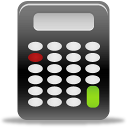# VAT Calculator

Updated on July 20, 2022

## VAT CalculatorMany retailers offer the value of VAT to their clients in the form of special promotions. Nonetheless, how do you figure out the VAT on a purchase that already includes the tax? Simply divide the price by 1.23 if you have a 23 percent interest rate, or by the appropriate amount if the rate is different. Simply enter the following as an example: if a computer costs \$590 with VAT, enter the following as an example: Is the promotion worth it?

If, on the other hand, you’re curious about the worth of a specific commodity but it hasn’t yet been charged with VAT, you can simply perform the opposite operation, i.e. multiply instead of divide. For example, if you wish to buy a tablet for \$320 excluding VAT, the calculation to use is:. If you’re still having trouble, you can compute the values in both scenarios using the two calculators listed below.

Calculate VAT (included in prices)

Price incl. VAT:  \$

Percentage of VAT:  %

### Calculate VAT (excluded in prices)

Price excl. VAT:  \$

Percentage of VAT:  %